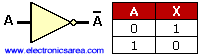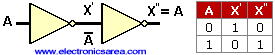# The logic NOT Gate

The logic NOT gate or Inverter Gate is a logic gate that has only one input and one output. The output logic level is the opposite of the input logic level. The symbol and the truth table of the NOT gate are shown bellow. This gate is represented by the following Boolean function: X = A’. Look at the picture and its truth table.

This means that if the input is a logical “1”, the output will be a logical “0” and if the input is a logical “0” the output will be a logical “1”. It implements a logical negation.Note: The apostrophe means “inverse” so A = X’ is equal to X = A.

The logic NOT gates can be connected in cascade, and the output of the second, fourth, sixth, etc. gate will be the same as the original input.One reason to use a circuit that has in its output the same of its input is to get a delay of the input signal.

•
•
•
•
•
•
•
•
•
•
•
•
•
•
•
•
•
•